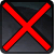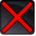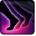# Energy ArcConditions

## Used by

Used by
• Class 16140997885803387191

## Related effects, buffs and debuffs

Please click on an effect below to view its details.

• [hidden] [1s]Effect #1

 Slot: Debuff Duration: 1s Tick rate: does not tick # occurrences: 0
• On Apply

Perform the following actions:

• Spell Damage
- Unknown (609) = (bool) false
- Damage Type = (int) 4
- Slot = (int) 1
- Spell Type = (int) 2
- Level Cap = (int) 0
- Amount Max = (float) 0
- Amount Min = (float) 0
- Amount Percent = (float) 0
- Threat Percent = (float) 0
- Standard Health Percent Max = (float) 0.0896
- Standard Health Percent Min = (float) 0.0896
- Amount Modifier Percent = (float) 0
- Coefficient = (float) 0
- Health Steal Percentage = (float) 0
• Play appearance epp . operation . oricon . fortress . boss . dread_master_brontes . tentacles . energy_sphere . energy_arc, dependent on calling effect
• [1.5s]Numbing Charge
Movement speed slowed by 10% per stack.

 Slot: Debuff Duration: 1.5s Tick rate: does not tick Initial stacks: 1 Max stacks: 5 # occurrences: 1 Tags: tag.​abl.​debuff.​snare Conditions: Can only by called by other effects
• When effect is applied

Only when the following conditions are met:

• If stacks of this effect = 1

Perform the following actions:

• Set movement speed to 90%
• When stacks of this effect increase

Only when the following conditions are met:

• If stacks of this effect = 2

Perform the following actions:

• Set movement speed to 80%
• When stacks of this effect increase

Only when the following conditions are met:

• If stacks of this effect = 3

Perform the following actions:

• Set movement speed to 70%
• When stacks of this effect increase

Only when the following conditions are met:

• If stacks of this effect = 4

Perform the following actions:

• Set movement speed to 60%
• When stacks of this effect increase

Only when the following conditions are met:

• If stacks of this effect = 5

Perform the following actions:

• Set movement speed to 50%
• When stacks of this effect increase

Only when the following conditions are met:

• If stacks of this effect = 6

Perform the following actions:

• Set movement speed to 40%
• When stacks of this effect increase

Only when the following conditions are met:

• If stacks of this effect = 7

Perform the following actions:

• Set movement speed to 30%
• When stacks of this effect increase

Only when the following conditions are met:

• If stacks of this effect = 8

Perform the following actions:

• Set movement speed to 20%
• When stacks of this effect increase

Only when the following conditions are met:

• If stacks of this effect = 9

Perform the following actions:

• Set movement speed to 10%
• When stacks of this effect increase
• When stacks of this effect increase

Only when the following conditions are met:

• If stacks of this effect = 10

Perform the following actions:

• Set movement speed to 0%